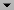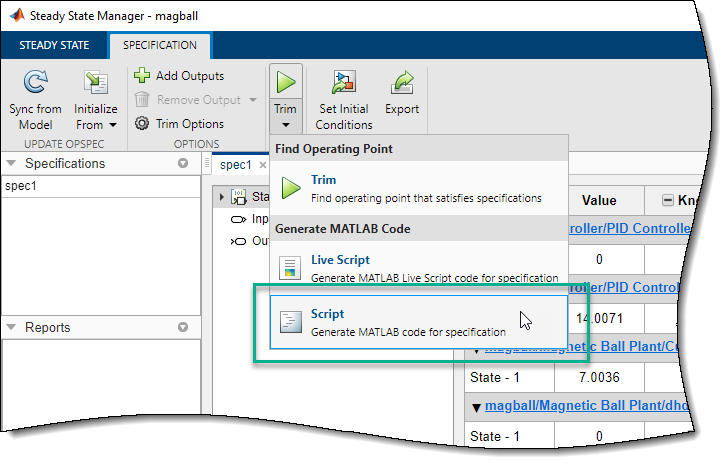Main Content

## Batch Compute Steady-State Operating Points Reusing Generated MATLAB Code

This example shows how to batch-compute steady-state operating points for a model using generated MATLAB® code. You can either simulate or linearize your model at these operating points and study the change in model behavior.

If you are new to writing scripts, interactively configure your operating points search using the Steady State Manager or Model Linearizer.

Before generating code for batch trimming, first compute an operating point to meet an instance of your specifications. For more information on computing operating points in:

After computing an operating point, generate a MATLAB script. To do so in the:

• In Steady State Manager, on the Specification tab, click Trim, and select `Script`.

• In Linear Analysis, in the Trim the model dialog box, click .

For more information on generating scripts, see Generate MATLAB Code for Operating Point Configuration.

The generated script opens in the MATLAB Editor window. You can then modify the script to trim the model at multiple operating points.

This example demonstrates batch trimming using the `magball` Simulink® model.

1. Open the model.

`open_system('magball')`
2. To open the Steady State Manager, in the Simulink model window, in the Apps gallery, click Steady State Manager.

3. On the Steady State tab, click Trim Specification.

4. In the spec1 document, in the Known column, select the magball/Magnetic Ball Plant/height state.5. Generate the trimming MATLAB code. On the Specification tab, click Trim, and select `Script`.6. In the MATLAB Editor window, modify the script to trim the model at multiple operating points.

1. Remove unneeded comments from the generated script.

2. Define the height variable, `height`, with values at which to compute operating points.

3. Add a `for` loop around the operating point search code to compute a steady-state operating point for each `height` value. Within the loop, before calling `findop`, update the reference ball height, specified by the Desired Height block.

Your script should look similar to the following code.

```%% Specify the model name model = 'magball'; %% Create the operating point specification object. opspec = operspec(model); %% Set the constraints on the states in the model. % State (5) - magball/Magnetic Ball Plant/height % - Default model initial conditions are used to initialize optimization. opspec.States(5).Known = true; %% Create the options opt = findopOptions('DisplayReport','iter'); %% Specify ball heights at which to compute operating points height = [0.05;0.1;0.15]; %% Loop over height values to find the corresponding operating points for i = 1:length(height) % Set the ball height in the specification. opspec.States(5).x = height(i); % Update the model ball haight reference parameter. set_param('magball/Desired Height','Value',num2str(height(i))) % Trim the model [op(i),opreport(i)] = findop(model,opspec,opt); end ```

After running this script, `op` contains operating points corresponding to each of the specified `height` values.

## SupportGet trial now